# Garden Hose Flow Rate and Time

The amount of water flow from a garden hose and minutes to supply that amount of water are determined below, based on the hose size and its supply pressure.

## Garden Hose Flow Rate Calculator

Hose Size:
in
Hose Supply Pressure:
psi
Hose Length:
feet
Water Flow Rate:

## Water Flow Time Calculator

Volume:
Time:

### The Equations

These calculators use these formulas to determine the water flow rate and time to fill a container with a garden hose.

#### Water Flow Rate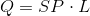Where: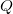= Water flow rate from the end of the hose (gpm)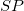= An arbitrary number derived from the hose size and supply pressure See Table.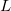= An arbitrary number to account for hose size See Table.

#### Water Flow Time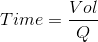Where:= Time it will take to fill the specified volume (min)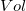= Volume needed (gal)= Flow rate from the garden hose (gpm)

To determine the value for, use this table.To determine the value for, use this table.

Length (ft)Value
254
502
751.5
1001
125.87
150.75
175.62
200.5
 Pressure (psi) Size (in) 40 45 50 60 1/2 6 6.5 7 7.5 5/8 11 12 12.5 14 3/4 18 19 20 22

Reference: Washington State University